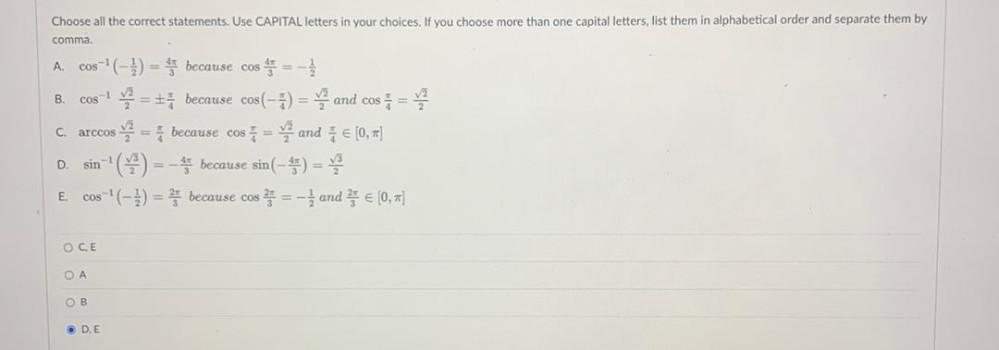Question:

# A B Choose all the correct statements. Use CAPITAL letters in your choices. If you choose more than one capital letters, list thA B Choose all the correct statements. Use CAPITAL letters in your choices. If you choose more than one capital letters, list them in alphabetical order and separate them by comma cos(-) = because cos * = -1 cost you + because cos(-1) = and cos = s me because cos = 2 and 3 € (0,#] sin = because sin(-5) = cos(-5) = 3 because cos=- and € (0,#] C. arccos D. E OCE OA OB ► DE